American Journal of Optics and Photonics
Volume 3, Issue 1, February 2015, Pages: 13-16

Study of the Electrons Density in the Base of the Parallel Vertical Junction Solar Cell Under the Influence of the Temperature

Nfally Dieme

Physics Department, Faculty of Science and Technology, Laboratory of Semiconductors and Solar Energy, University Cheikh Anta Diop, Dakar, Senegal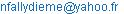Nfally Dieme. Study of the Electrons Density in the Base of the Parallel Vertical Junction Solar Cell Under the Influence of the Temperature. American Journal of Optics and Photonics. Vol. 3, No. 1, 2015, pp. 13-16. doi: 10.11648/j.ajop.20150301.13

Abstract: This work is a theoretical study of a parallel vertical junction solar cell under multispectral illumination in static regime. The expression for the density of the minority carriers in excess (electrons) in the base is determined from the equation of continuity. It's an equation that takes into account the process of generation of the charge carriers, the process of diffusion of these carriers and their processes of recombination process. The aim of this work is to study the evolution of this density depending on temperature and its distribution in depth following x and the thickness z in the base for different values of the temperature.

Keywords: Vertical Junction, Electron’S Density, Temperature, Thickness z – Base, Depth x

1 Introduction

The operation of a photovoltaic cell is based on the interaction between a photon and an electron of the semiconductor. An important part of incoming solar radiation (about 84%) arriving at the solar cells is stored in the form of heat. And it creates an increase in the temperature prevailing in the material . This appears high if this heat is not evacuated.

The aim of this work is to investigate the influence of temperature on carrier's density.

In the first part of this work, we are going to determine the excess minority carrier's density from continuity equation and in the second part, present our simulation results on evolution of this density depending on temperature and its distribution in depth following x and the thickness z in the base for different values of the temperature.

2. Mathematical Study

2.1. Hypotheses

This study is based on a vertical parallel junction silicon solar cell  presented on figure 1. The solar cell is illuminated along the z axis in steady state.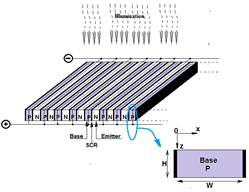Fig. 1. Vertical parallel junction solar cell (H =0,02cm; W =0,03cm).

We assume that the following hypotheses are satisfied.

The contribution of the emitter is neglected.

Illumination is made with polychromatic light, and is considered to be uniform on the z = 0 plane.

There is no electric field without space charge regions.

2.2. Electrons Density

When the solar cell is illuminated, there are simultaneously three major phenomena that happen: generation diffusion and recombination.

These phenomena are described by the diffusion- recombination equation given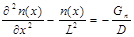(1)

D is the diffusion constant and is related to the operating temperature through the relation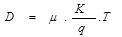(2)

with q the elementary charge, k the Boltzmann constant and T the temperature.

Gn= g(z)+gth is the carrier generation rate.

g(z) is the carrier generation rate at the depth z in the base and can be written as :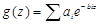(3)

ai and bi are obtained from the tabulated values of AM1.5 solar illumination spectrum and the dependence of the absorption coefficient of silicon with illumination wavelength.

gth is the thermal generation rate. But in the absence of temperature gradient gth is uniformity compensated by the thermal recombination rate ,

It is given by: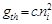(4)

with(5)

ni refers to the intrinsic concentration of minority carriers in the base, An is a specific constant of the material (An=3.87x1016 for silicon) and Nb is the base doping concentration in impurity atoms.

And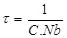(6)

n(x), L, t, and μ are respectively the excess minority carriers density, diffusion length, lifetime and mobility and C is the proportionality coefficient.

The solution of equation (1) is: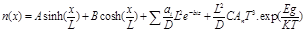(7)

Coefficients A and B are determined through the following boundary conditions at the junction (x=0) , , :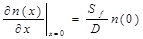(8)

This boundary condition introduces a parameter Sf which is called recombination velocity at the junction. Sf determines the charge carriers flow through the junction and is directly related to the operating point of the solar cell 

The higher Sf is, the higher the current density will be.

in the middle of the base (x=W/2) ,  :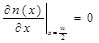( 9)

3. Results and Discussion

The height of the gap or the energy gap (Eg) plays an important role in the interaction of a semiconductor with light radiation. This height strongly depends on the temperature; , .

The following figure shows the evolution of Eg depending on the temperature.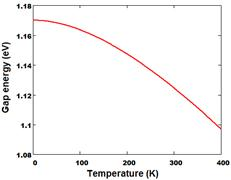Fig. 2. Energy gap versus temperature.

When a solar cell is subjected to illumination an important fraction of the incident energy is stored as heat. The heat creates a positive temperature gradient in the material. The rise in temperature causes a decrease of the energy gap , 

3.1. Evolution of the Electrons Density Depending on the Temperature

The following figure illustrates the evolution of the density of these carriers (electrons) with the temperature.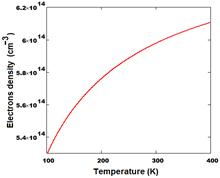Fig. 3. Excess minority carriers density in the base versus temperature. x=10-3cm, z=10-3cm, Sf=100cm.s-1.

Figure 3 shows the evolution of density of the charge carriers minority in excess in the base (electrons), depending on the temperature prevailing on average in the base.

We note that the carrier density increases when the temperature increases.

Indeed when the photocell is enlightened, much of the incident energy is stored as heat in the cell. The increase in temperature reduces the height of the band gap of the material .

Thus the solar cell becomes sensitive to the excitement of the wide band of wavelengths of the light spectrum . And therefore many electrons are easily extracted from the valence band and pass into the conduction band. This photogeneration is accompanied by thermalagitation due to the increase in temperature, and which also ejects electrons in the conductionband. This carrier generation process caused by the temperature is called thermogeneration .

3.2. Distribution of the Electrons Density Following the Depth X in the Base

The distribution of the density of the charge carriers following the depth x in the base is illustrated by Figure4 below.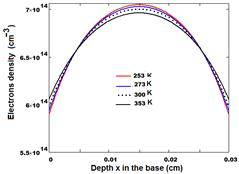Fig. 4. Electron density versus depth x in the base for various temperatures. z=10-3cm, Sf=100cm.s-1.

The figure. 4 shows that:

For a given temperature, the density of the charge carriers (electrons) is maximum in the middle of the base and decreases when we approach junctions,

at the centre of the base, the density of the charge carriers decreases when the temperature increases.

There are depths in the base from which, observed an inverse situation compared to the center of the base. Everything looks as if in these levels a storage of charge carriers begin and which intensifies when the temperature increases.

All this can be explained by the theory of the photoelectric effect. The lot electrons is photogenerated with a large kinetic energy due to the reducing of the height of the band gap caused by the increase in temperature. Therefore they start moving. And the electrons in excess, more will leave, (when the temperature is high), the center of the base where they are heavily generated to move the joints where they will be strongly stored.

3.3. Distribution of the Density of the Charge Carriers Following the Thickness z of the Base

The figure5 shows the distribution of the density of the charge carriers following the thickness z of the base.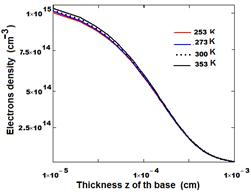Fig. 5. Electrons density versus thickness z of the base for various temperatures x= 10-3cm, Sf=100cm.s-1.

Figure5 shows that the excess minority carriers density in the base, decreases depending on the thickness of the base. This decrease is even more important that the temperature is low.

This simple constant allows us to highlight the generation of charge bulk carriers. In other words the photoelectric effect is not only surface, it is also volume. There is therefore some photons which penetrates deeply into the material and cause electron extraction. But it has less generation of electrons in volume than in surface exposed to sunlight. High volume recombinations due by the defects in structuring and traps center for photogenerated electrons are so important that the electron density remains low. Moreover high thickness retains heat for a long time and the solar cell considerably loses its ability to store charge carriers at the junction with increasing temperature

4. Conclusion

In the present work we have done a study of a solar cell simulation parallel vertical junction. We have shown that temperature strongly contributes to the generation of the charge carriers.

The carrers photogenerated under the effect of temperature are equipped with a high kinetic energy and start moving. The density of electrons in the base is maximum in the middle and decreases depending on thickness. This study can be confirmed by studying the solar cell electrical parameters under the influence of thickness

References

1. K. Agroui, Etude du Comportement Thermique de Modules Photovoltaïques de Technologie Monoverre et Biverre au Silicium Cristallin, Rev. Energ. Ren. : Valorisation, pp. 7-11, (1999).
2. Nf. Dieme, et al., Influence of Temperature on the Electrical Parameters of a Vertical Parallel Junction Silicon Solar Cell under Polychromatic Illumination in Steady State, Res.J. App. Sci., Eng. and Technology, vol. 7, 2559-2562,( 2014)
3. Francis Levy, Traité des matériaux 18: Physique et technologie des semi-conducteurs, Presses Polytechniques et Universitaires Romandes, (1995).
4. Ly Diallo, H., B. Dieng, I. Ly, M.M. Dione, A. Wereme, M. Ndiaye and G. Sissoko, Determination of the recombination and electrical parameters of a vertical multijunction silicon solar cell. Res. J. Appl. Sci. Eng. Technol., 4 2626-2631. (2012)
5. A. Thiam, M. Zoungrana, H. Ly Diallo, A Diao, N. Thiam, S. Gueye, M.M. Deme, M. Sarr and G. Sissoko, Influence of Incident Illumination Angle on Capacitance of a Silicon Solar Cell under Frequency Modulation, Res.J. App. Sci., Eng. and Technology, 5 1123-1128 (2013)
6. Dieme Nf study of the performance of a parallel vertical junction Silicon solar cell under thermal influence, Asian Academic Research Journal of Multidisciplinary, 2 2319 – 280. (2015)
7. M. Sane, M. Zoungrana, H. L. Diallo, G. Sahin, N. Thiam,M. Ndiaye, M. Dieng, G. Sissoko, Influence of Incidence Angle on the Electrical Parameters of a vertical Silicon Solar Cell under Frequency Modulation, International Journal of Inventive Engineering and Sciences (IJIES) 1 2319–9598. (2013)
8. S. M. Sze, Kwok K. Ng, Physics of Semiconductor Devices, Third Edition, John Wiley & Sons, (2007).
9. Roland Pässler, Semi-empirical descriptions of temperature dependences of band gaps in semiconductors, phys. stat. sol. vol 3,, pp.710–728 (2003)
10. S. Valkov, Electronique analogique, Edition Castéilla, Collection A.CAPLIEZ, (1994).

 Contents 1 2. 2.1. 2.2. 3. 3.1. 3.2. 3.3. 4.
Article ToolsAbstractPDF(227K)